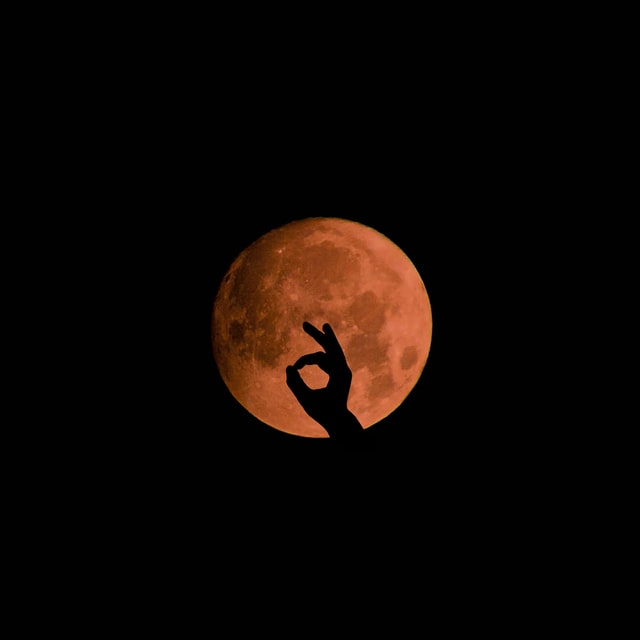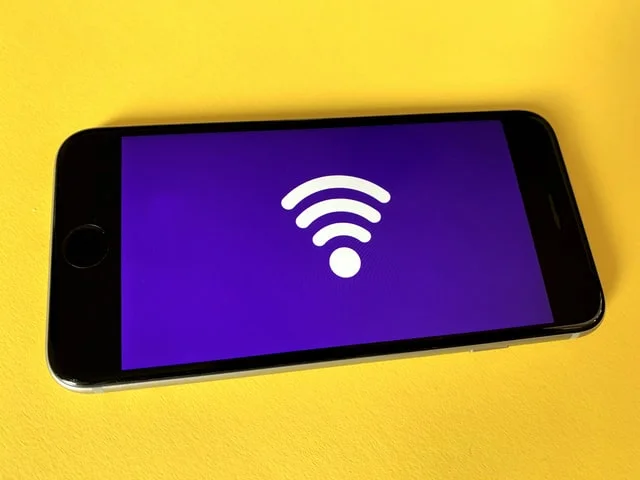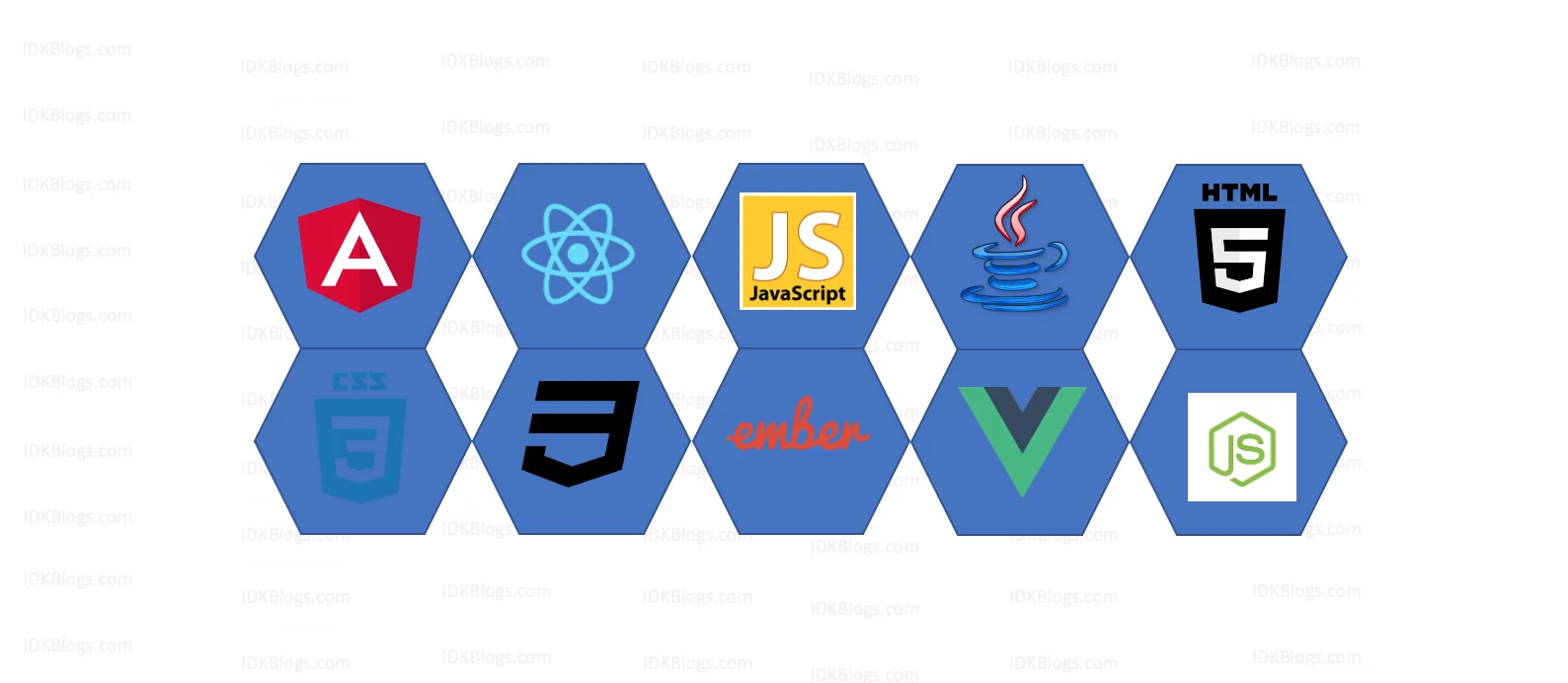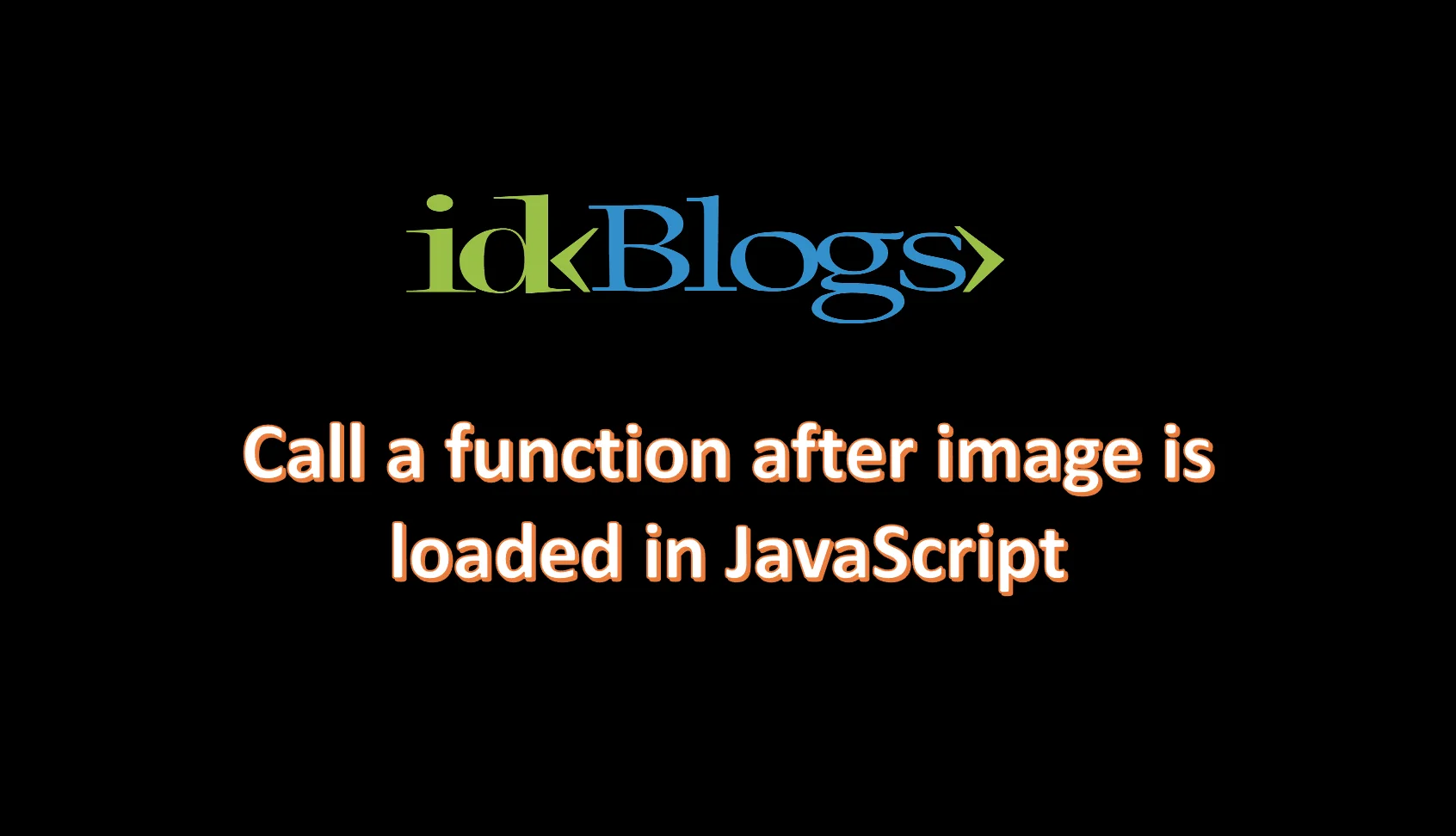# Convert date to milliseconds in javascript

### How can I convert given ISO date into milliseconds?

idkblogs.com      August 29, 2020Shubham VermaS

ometime we need to compare the two date in our project or we need to store the date into db, then we need to convert this date into milliseconds. Because storing milliseconds in db is very easy and converting date into milliseconds is also very easy. In this article we\'ll see how we can convert the given dates to milliseconds.

### Let's see the ways how we can convert the date to milliseconds:

The number of milliseconds elapsed from January 1, 1970. If your date is less than that date, the value will be negative

``````                          var date = new Date("08/30/2020 16:00:00");
var milliseconds = date.getTime();
console.log(milliseconds);
``````

``````                          var date = new Date("08/30/2020");
var milliseconds = date.getTime();
console.log(milliseconds);
``````

``````                          var date = new Date();
var milliseconds = date.getTime();
console.log(milliseconds);
``````

``````                          var date = new Date("08/30/1960 16:00:00");
var milliseconds = date.getTime();
console.log(milliseconds);
``````Convert date to milliseconds in javascript

``````                          var date = new Date();
var myDate = Date.parse(date);
console.log(myDate);
``````

``````                          var date = new Date('08/30/2020');
var myDate = Date.parse(date);
console.log(myDate);
``````

Thank you

To read more about the technologies, Please subscribe us, You'll get the monthly newsletter having all the published article of the last month.

### OTHER POSTS